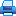Address: office 1410a, 17 Naberezhnaya Severnoy Dviny, Arkhangelsk, 163002, Russian Federation, Northern (Arctic) Federal University named after M.V. Lomonosov

Phone: (818-2) 21-61-21
E-mail: vestnik_est@narfu.ru
http://aer.narfu.ru/en/

# Mathematical Modelling of Transport Processes in the Problem of Poiseuille Flow on the Basis of Williams Equation. Pp. 84–89.Section: Physics. Mathematics. Informatics

533.72

### Authors

Gulakova Svetlana Viktorovna, Postgraduate Student, Institute of Mathematics, Information and Space Technologies, Northern (Arctic) Federal University named after M.V. Lomonosov (Arkhangelsk, Russia)

Popov Vasily Nikolaevich, Institute of Mathematics, Information and Space Technologies, Northern (Arctic) Federal University named after M.V. Lomonosov (Arkhangelsk, Russia)

### Abstract

The paper provides an analytical solution to the problem of Poiseuille flow using the kinetic approach. As the basic equation we used the linearized Williams equation, and as a boundary condition on the channel walls we used a diffuse reflection model. The structure of mass rate of gas and mass flux per unit of channel width were calculated. The results were compared with the analogous data obtained by numerical methods.

### Keywords

Boltzmann equation, model kinetic equations, Poiseuille flow, exact analytical solution.

The full-text version of the article can be requested through the university’s library.

### References

1. Koshmarov Yu.A., Ryzhov Yu.A. Prikladnaya dinamika razrezhennogo gaza [Applied Dynamics of Rarefied Gas]. Moscow, 1977. 184 p.
2. Latyshev A.V., Yushkanov A.A. Kineticheskie uravneniya tipa Vil’yamsa i ikh tochnye resheniya [Williams Kinetic Equations and Their Exact Solutions]. Moscow, 2004. 271 p.
3. Popov V.N., Testova I.V., Yushkanov A.A. Analiticheskoe reshenie zadachi o techenii Puazeylya s ispol’zovaniem ellipsoidal’no-statisticheskoy modeli kineticheskogo uravneniya Bol’tsmana [Analytical Solution of the Poiseuille Flow Problem Using the Ellipsoidal-Statistical Model of the Boltzmann Kinetic Equation]. Prikladnaya mekhanika i tekhnicheskaya fizika, 2012, no. 4, pp. 48–56.
4. Popov V., Testova I., Yushkanov A.A. Matematicheskoe modelirovanie techeniy gaza v kanalakh [Mathematical Modeling of Gas Flows in Channels]. Saarbrucken, 2012. 116 p.
5. Loyalka S.K., Hickey K.A. Plane Poiseuille Flow: Near Continuum Results for a Rigid Sphere Gas. Physica A, 1989, vol. 160, no. 3, pp. 395–408.
6. Siewert C.E. Poiseuille, Thermal Creep and Couette Flow: Results Based on the CES Model of the Linearized Boltzmann Equation. European Journal of Mechanics – B/Fluids, 2002, vol. 21, pp. 579–497.
7. Siewert C.E., Garcia R.D.M., Grandjean P. A Concise and Accurate Solution for Poiseuille Flow in a Plane Channel. Journal of Mathematical Physics, 1980, vol. 21, pp. 2760–2763.